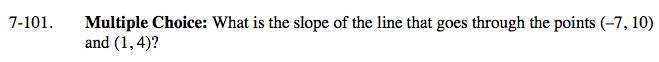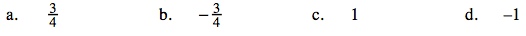Home > CAAC > Chapter 7 > Lesson 7.3.2 > Problem7-101

7-101.
1. Multiple Choice: What is the slope of the line that goes through the points (−7, 10) and (1, 4)? Homework Help ✎

1.2.3. 1

4. −1Graph the two points and make a slope triangle to find the slope.

(b)